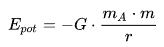# Nuclear EnergyNuclear Power Plant Isar, GermanySpent nuclear fuel poolTurbine of a nuclear plant

# Potential energyIn physics, the potential energy is the energy that an object possesses due to its position in a force field or that a system has due to the configuration of its parts.

There are many types of potential energy, but the most common are:

• Gravitational potential energy that depends on the vertical position and the mass of an object.
• Elastic potential energy of an extended spring
• Electric potential energy of a charge in an electric field.

The unit of measurement of the International System of Units for energy is the joule (J).

Potential energy is associated with the forces acting on a body in such a way that this only depends on the position of the body in space; these forces can be represented by a vector at any point in space forming what is known as a vector field of forces or field of forces.

If the work of a force field acting on a body moving from an initial position to a final position is determined only by these two positions, and therefore, it does not depend on the trajectory of the body - then there is a function called Potential energy that can be evaluated at the two positions to determine the work.

The term "potential energy" was introduced by nineteenth-century Scottish engineer and physicist William Rankine, although it is related to the Greek philosopher Aristotle's concept of potentiality.

## Gravitational potential energy

The gravitational potential energy is the potential energy that an object gains by the gravitational effect of another object (usually very large bodies, for example, the Earth, the Sun). This energy is directly proportional to the mass of the object and the size of the mass attracted. The magnitude of the mass attracted creates the acceleration of gravity. This acceleration for the world is approximately 9.8 m / s² on the surface of the earth.

Because there are different types of forces, there are also different types of potential energy. The potential energy as a result of the force of gravity, for example, is called gravitational energy or potential gravitational energy. For an object with mass m, at height h, the following expression is applied to the object's gravitational energy:

Epot = m · g · h

Where (in SI units):

Epot - The potential energy in joules (J).
M - the mass in kilograms (kg)
h - the height in meters (m)
g - the acceleration of fall in m / s² (approximately 9.81 m / s²)

The potential energy if the object is in the level. h = 0 is set to zero.

However, when this object is located a great distance from the surface of the earth, gravity is no longer constant and the previous formula no longer applies.

The expression of potential gravitational energy then becomes:The potential energy at an infinite distance from Earth is here to zero, because the formula is the simplest (this is because, unlike many other cases, the energy difference here has a finite limit). The potential energy is, therefore, always negative, and as in the model mentioned above, it increases as the object moves away from the ground.

## Transformation of potential energy to other types of energy

Energy can manifest itself in many forms, including chemical energy, thermal energy, electromagnetic radiation, gravitational energy, electrical energy, elastic energy, nuclear energy, and energy from the rest. All these types of energy can be classified into two main classes: potential energy and kinetic energy. Potential energy can be transferred between objects and transformed into other types of energy.

### Example of transformation of potential energy

The potential energy can be transformed into other types of energy such as, for example, the kinetic energy that can be easily illustrated by the following example:

By kicking a ball the player transmits a potential energy of his leg to the ball, which becomes potential elastic energy by deforming the ball and compressing the air inside. By regaining the original shape, this elastic potential energy of the ball is converted into kinetic energy at the moment when the ball leaves at full speed.

If the player has kicked the ball with a completely vertical trajectory, as the ball loses speed it will lose kinetic energy, which will turn into gravitational potential energy. This will happen until reaching the highest point, at this time, the ball will not have kinetic energy and all its energy will be potential. When the balloon begins to fall, the gravitational potential energy reverts to kinetic energy.

valoración: 3 - votos 1

Last review: September 1, 2017

Back